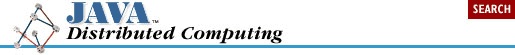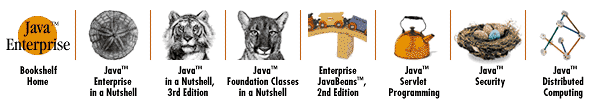home | O'Reilly's CD bookshelfs | FreeBSD | Linux | Cisco | Cisco Exam## 3.4. Distributed Object Schemes for Java

While there are several distributed object schemes that can be used within the Java environment, we'll only cover two that qualify as serious options for developing your distributed applications: CORBA and RMI. Both of them have their advantages and their limitations, which we'll look at in detail in the following sections.

During this discussion, we'll be using an example involving a generic problem solver, which we'll distribute using both CORBA and RMI. We'll show in each case how instances of this class can be used remotely using these various object distribution schemes. A Java interface for the example class, called Solver, is shown in Example 3-1. The Solver acts as a generic compute engine that solves numerical problems. Problems are given to the Solver in the form of ProblemSet objects; the ProblemSet interface is shown in Example 3-2. The ProblemSet holds all of the information describing a problem to be solved by the Solver. The ProblemSet also contains fields for the solution to the problem it represents. In our highly simplified example, we're assuming that any problem is described by a single floating-point number, and the solution is also a single floating-point value.

#### Example 3-1. A Problem Solver Interface

```package dcj.examples;

import java.io.OutputStream;

//
// Solver:
// An interface to a generic solver that operates on ProblemSets
//

public interface Solver
{
// Solve the current problem set
public boolean solve();

// Solve the given problem set
public boolean solve(ProblemSet s, int numIters);

// Get/set the current problem set
public ProblemSet getProblem();
public void setProblem(ProblemSet s);

// Get/set the current iteration setting
public int getInterations();
public void setIterations(int numIter);

// Print solution results to the output stream
public void printResults(OutputStream os);
}```

#### Example 3-2. A Problem Set Class

```package dcj.examples;

public class ProblemSet
{
protected double value = 0.0;
protected double solution = 0.0;

public double getValue() { return value; }
public double getSolution() { return solution; }
public void setValue(double v) { value = v; }
public void setSolution(double s) { solution = s; }
}```

Our Solver interface represents a pretty simple compute engine, but it has some features meant to highlight the attributes of a distributed object scheme. As we said before, the Solver accepts problems in the form of ProblemSet objects. It also has a single compute parameter, the number of iterations used in solving the problem. Most computational algorithms have parameters that can be used to alter the way a problem is solved: basic iterative methods usually have a maximum number of iterations to run, so we're using that as the only parameter on our simplified Solver.

A Solver has two solve() methods. One has no arguments and causes the Solver to solve the default problem using the default settings. The default problem for the Solver can be set using the setProblem() method, and the default iteration limit can be set using the setIterations() method. You can also get these values using the getProblem() and getIterations() methods on the interface. The other solve() method includes arguments that give the problem to be solved, and the iteration limit to use for solving the problem.

This Solver interface acts as a sort of litmus test for distributed object schemes. It includes methods that accept Object arguments (ProblemSets, specifically), it can maintain its own state (the default problem and default iteration limit), which needs to be kept consistent across method calls from multiple clients, and it includes a method that involves generic I/O. We'll see in the following examples how well both CORBA and RMI support remote implementation of this interface.